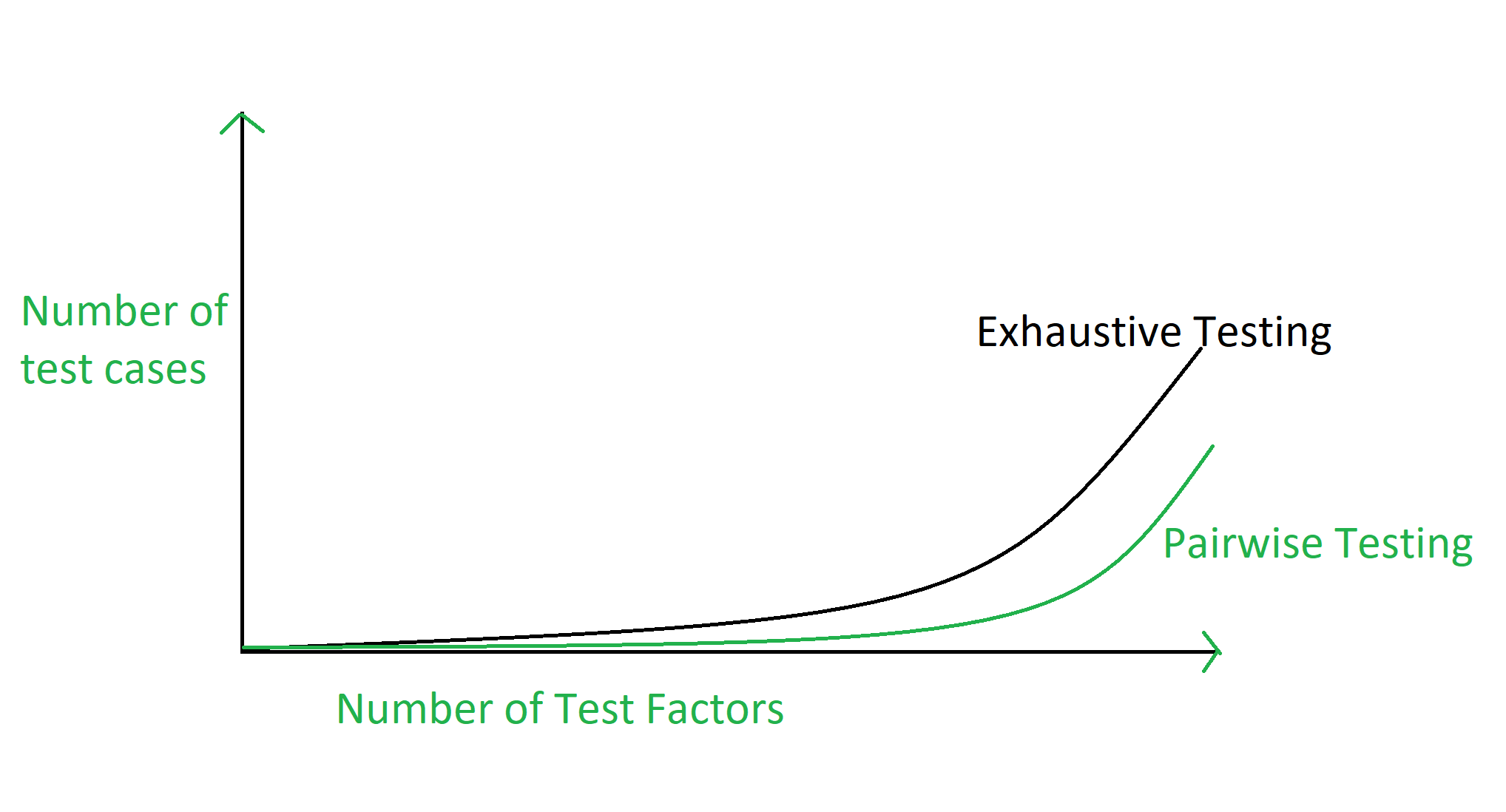# Pairwise Software Testing

Pairwise Testing is a type of software testing in which permutation and combination method is used to test the software. Pairwise testing is used to test all the possible discrete combinations of the parameters involved.

Pairwise testing is a P&C based method, in which to test a system or an application, for each pair of input parameters of a system, all possible discrete combinations of the parameters are tested. By using the conventional or exhaustive testing approach it may be hard to test the system but by using the permutation and combination method it can be easily done.

Example:
Suppose there is a software to be tested which has 20 inputs and 20 possible settings for each input so in that case there are total 20^20 possible inputs to be tested. Therefore in this case, exhaustive testing is impossible even all combinations are tried to be tested.

Graphical Representation of Pairwise Testing:Generalized form of Pairwise Testing:
The generalized form of pairwise testing is N-wise testing. Basically sorting is applied to the set,

```X = n{i},
so that P = P{i} gets ordered too. ```

Let the sorted set be a N tuple:

```P{s} = { P{i} } ;  i  |R(P{i})| < |R(P{j})|
Now take the set X(2) = { P{N-1}, P{N-2} } ```

And call it the pairwise testing. Generalizing further take the set

`X(3) = { P{N-1}, P{N-2}, P{N-3} }`

And call it the 3-wise testing. Similarly, we can say,

`X(K) = { P{N-1}, P{N-2}, ..., P{N-K} } `

K-wise testing.
The N-wise testing is all possible combinations from the above formula.

The advantages of pairwise testing are:

• Pairwise testing reduces the number of execution of test cases.
• Pairwise testing increases the test coverage almost up to hundred percentage.
• Pairwise testing increases the defect detection ratio.
• Pairwise testing takes less time to complete the execution of the test suite.
• Pairwise testing reduces the overall testing budget for a project.

The disadvantages of pairwise testing are:

• Pairwise testing is not beneficial if the values of the variables are inappropriate.
• In pairwise testing it is possible to miss the highly probable combination while selecting the test data.
• In pairwise testing, defect yield ratio may be reduced if a combination is missed.
• Pairwise testing is not useful if combinations of variables are not understood correctly.

My Personal Notes arrow_drop_upCheck out this Author's contributed articles.

If you like GeeksforGeeks and would like to contribute, you can also write an article using contribute.geeksforgeeks.org or mail your article to contribute@geeksforgeeks.org. See your article appearing on the GeeksforGeeks main page and help other Geeks.

Please Improve this article if you find anything incorrect by clicking on the "Improve Article" button below.

Article Tags :

Be the First to upvote.

Please write to us at contribute@geeksforgeeks.org to report any issue with the above content.